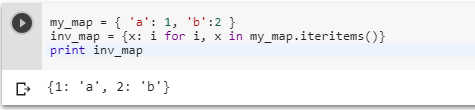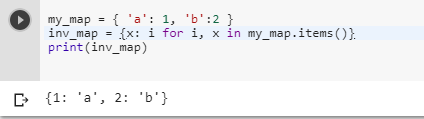2 views
in Python

Given a dictionary like so:

my_map = { 'a': 1, 'b':2 }

How can one invert this map to get:

inv_map = { 1: 'a', 2: 'b' }

by (106k points)

To do reverse / invert a mapping in Python 2.7.x you can use the following piece of code:-

my_map = { 'a': 1, 'b':2 }

inv_map = {x: i for i, x in my_map.iteritems()}

Print inv_mapIf you want to do reverse / invert a mapping you can use the following piece of code:-

my_map = { 'a': 1, 'b':2 }

inv_map = {x: i for i, x in my_map.items()}

print(inv_map)+1 vote